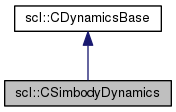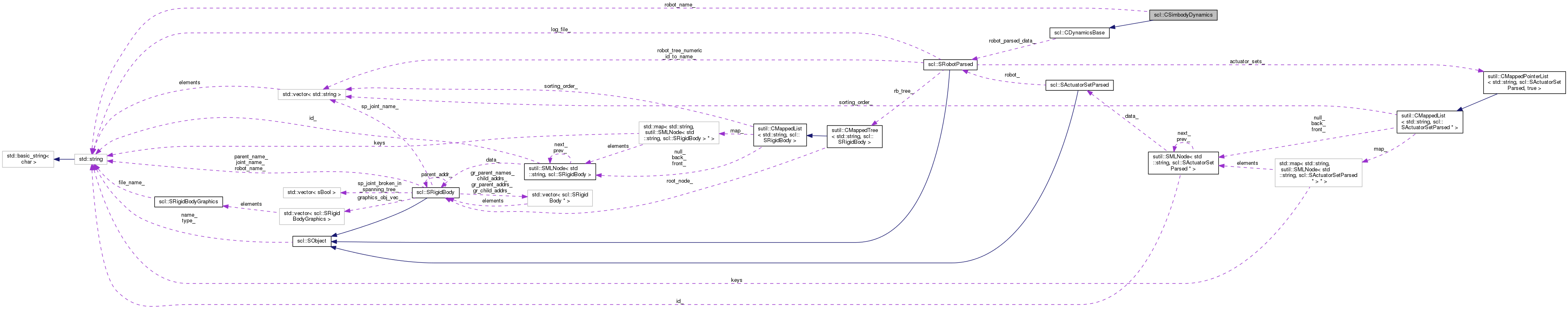SCL  1.0 Standard Control Library : Control, dynamics, physics, and simulation
scl::CSimbodyDynamics Class Reference

#include <CSimbodyDynamics.hpp>

Inheritance diagram for scl::CSimbodyDynamics:[legend]
Collaboration diagram for scl::CSimbodyDynamics:[legend]

Public Member Functions

virtual bool init (const SRobotParsed &arg_robot_data)

virtual bool computeGCModel (SRobotSensors const *sensor_data, SGcModel *js_model)

virtual sBool computeTransform_Depracated (const void *arg_link_id, Eigen::Affine3d &arg_T)

virtual sBool computeJacobian_Depracated (const void *arg_link_id, const Eigen::VectorXd &arg_pos_global, Eigen::MatrixXd &arg_J)

virtual sBool integrate (SRobotIO &arg_inputs, const sFloat arg_time_interval)

virtual sFloat getKineticEnergy_Depracated ()

virtual sFloat getPotentialEnergy_Depracated ()

CSimbodyDynamics ()

virtual ~CSimbodyDynamics ()

virtual sBool computeTransformsForAllLinks (sutil::CMappedTree< std::string, SRigidBodyDyn > &arg_tree, const Eigen::VectorXd &arg_q)

virtual sBool computeTransform (SRigidBodyDyn &arg_link, const Eigen::VectorXd &arg_q)

virtual sBool computeTransformToAncestor (Eigen::Affine3d &arg_T, SRigidBodyDyn &arg_link, const SRigidBodyDyn *arg_ancestor, const Eigen::VectorXd &arg_q)

virtual sBool computeJacobianWithTransforms (Eigen::MatrixXd &arg_J, SRigidBodyDyn &arg_link, const Eigen::VectorXd &arg_q, const Eigen::Vector3d &arg_pos_local)

virtual sBool computeJacobian (Eigen::MatrixXd &arg_J, const SRigidBodyDyn &arg_link, const Eigen::VectorXd &arg_q, const Eigen::Vector3d &arg_pos_local) const

virtual sBool computeJacobian (Eigen::MatrixXd &arg_J, const SRigidBodyDyn &arg_link, const SRigidBodyDyn *arg_ancestor, const Eigen::VectorXd &arg_q, const Eigen::Vector3d &arg_pos_local)

virtual sBool computeJacobianTrans (Eigen::MatrixXd &arg_J, const SRigidBodyDyn &arg_link, const SRigidBodyDyn *arg_ancestor, const Eigen::VectorXd &arg_q, const Eigen::Vector3d &arg_pos_local)

virtual sBool computeJacobianRot (Eigen::MatrixXd &arg_J, const SRigidBodyDyn &arg_link, const SRigidBodyDyn *arg_ancestor, const Eigen::VectorXd &arg_q, const Eigen::Vector3d &arg_pos_local)

virtual sBool computeExternalContacts (sutil::CMappedList< std::string, SForce > &arg_contacts)

virtual sFloat computeEnergyKinetic (sutil::CMappedTree< std::string, SRigidBodyDyn > &arg_tree, const Eigen::VectorXd &arg_q, const Eigen::VectorXd &arg_dq)

virtual sFloat computeEnergyPotential (sutil::CMappedTree< std::string, SRigidBodyDyn > &arg_tree, const Eigen::VectorXd &arg_q)

virtual sBool hasBeenInit ()

Protected Attributes

sBool has_been_init_

const SRobotParsedrobot_parsed_data_

Private Attributes

std::string robot_name_

size_t ndof_

Eigen::Vector3d gravity_

SimTK::MultibodySystem simbody_system_

SimTK::SimbodyMatterSubsystem simbody_matter_

SimTK::GeneralForceSubsystem simbody_forces_

SimTK::RungeKuttaMersonIntegrator * simbody_rkm_integ_

SimTK::TimeStepper * simbody_ts_

SimTK::State simbody_state_

Detailed Description

Computes the dynamics of a robot model using the Simbody dynamics engine

Constructor & Destructor Documentation

 scl::CSimbodyDynamics::CSimbodyDynamics ( )

Constructor : Sets defaults

 scl::CSimbodyDynamics::~CSimbodyDynamics ( )
virtual

Destructor : Deletes stuff

Member Function Documentation

private

 virtual sFloat scl::CDynamicsBase::computeEnergyKinetic ( sutil::CMappedTree< std::string, SRigidBodyDyn > & arg_tree, const Eigen::VectorXd & arg_q, const Eigen::VectorXd & arg_dq )
inlinevirtualinherited

Gets the robot's kinetic energy

Parameters
 arg_tree The tree for which the transformation matrices are to be updated arg_q The current generalized coordinates. arg_dq The current generalized velocities.

Reimplemented in scl::CDynamicsScl, scl_ext::CDynamics3d, and scl_ext::CDynamicsSclSpatial.

 virtual sFloat scl::CDynamicsBase::computeEnergyPotential ( sutil::CMappedTree< std::string, SRigidBodyDyn > & arg_tree, const Eigen::VectorXd & arg_q )
inlinevirtualinherited

Gets the robot's potential energy

Parameters
 arg_tree The tree for which the transformation matrices are to be updated arg_q The current generalized coordinates.

Reimplemented in scl::CDynamicsScl, scl_ext::CDynamicsSclSpatial, and scl_ext::CDynamics3d.

 virtual sBool scl::CDynamicsBase::computeExternalContacts ( sutil::CMappedList< std::string, SForce > & arg_contacts )
inlinevirtualinherited

Calculates the collision forces for the robot to which this dynamics object is assigned.

Uses std::string based force lookup. The dynamics implementation should support this (maintain a map or something).

NOTE : The dynamics engine may delete forces from the passed mapped list at will. Do not store pointers to them at random places in your code.

As of now, this is optional for dynamics engines

Parameters
 arg_contacts This is where the simulator will store the contact forces. It may use the std::string to identify when to remove or re-add forces.

Reimplemented in scl_ext::CDynamics3d.

 bool scl::CSimbodyDynamics::computeGCModel ( SRobotSensors const * arg_sensor_data, SGcModel * arg_gc_model )
virtual

Updates the generalized coordinate model matrices (Everything in SGcModel).

This is the most efficient method to access the standard matrices. Computing transformations and Jacobians individually is typically wasteful.

Parameters
 arg_sensor_data This is where there current robot state is read when updateModelMatrices() is called. arg_gc_model Pointer to the joint-space model structure for the robot. This is the place where the matrices reside that will get updated when calling updateModelMatrices().

Implements scl::CDynamicsBase.

 virtual sBool scl::CDynamicsBase::computeJacobian ( Eigen::MatrixXd & arg_J, const SRigidBodyDyn & arg_link, const Eigen::VectorXd & arg_q, const Eigen::Vector3d & arg_pos_local ) const
inlinevirtualinherited

Calculates the Jacobian for the robot to which this dynamics object is assigned. dx_global_origin = Jx . dq The Jacobian is specified by a link and an offset (in task space dimensions)from that link. ** CONST VERSION : NOTE : The regular version should call this. **

Parameters
 arg_J The Jacobain will be saved here. arg_link The link at which the Jacobian is to be calculated arg_q The current generalized coordinates. arg_pos_local The offset from the link's frame (in link coordinates).

Reimplemented in scl::CDynamicsScl.

 virtual sBool scl::CDynamicsBase::computeJacobian ( Eigen::MatrixXd & arg_J, const SRigidBodyDyn & arg_link, const SRigidBodyDyn * arg_ancestor, const Eigen::VectorXd & arg_q, const Eigen::Vector3d & arg_pos_local )
inlinevirtualinherited

Calculates the Jacobian for the robot to which this dynamics object is assigned. dx_ancestor_coords = Jx . dq The Jacobian is specified by a link and an offset (in task space dimensions)from that link.

Parameters
 arg_J The Jacobain will be saved here. arg_link The link at which the Jacobian is to be calculated arg_ancestor The link up to which the Jacobian is to be calculated Pass NULL to compute the Jacobian up to the global root. arg_q The current generalized coordinates. arg_pos_local The offset from the link's frame (in link coordinates).
 sBool scl::CSimbodyDynamics::computeJacobian_Depracated ( const void * arg_link_id, const Eigen::VectorXd & arg_pos_global, Eigen::MatrixXd & arg_J )
virtual

Calculates the Jacobian for the robot to which this dynamics object is assigned.

Uses id based link lookup. The dynamics implementation should support this (maintain a map or something).

Parameters
 arg_link_id The link at which the Jacobian is to be calculated arg_pos_global The offset from the link's frame. arg_J The Jacobain will be saved here.
 virtual sBool scl::CDynamicsBase::computeJacobianRot ( Eigen::MatrixXd & arg_J, const SRigidBodyDyn & arg_link, const SRigidBodyDyn * arg_ancestor, const Eigen::VectorXd & arg_q, const Eigen::Vector3d & arg_pos_local )
inlinevirtualinherited

Calculates the Jacobian for the robot to which this dynamics object is assigned. ONLY for rotation. Ie. size(Jx) = (3,ndof) dx_ancestor_coords = Jx . dq

Parameters
 arg_J The Jacobain will be saved here. arg_link The link at which the Jacobian is to be calculated arg_ancestor The link up to which the Jacobian is to be calculated Pass NULL to compute the Jacobian up to the global root. arg_q The current generalized coordinates. arg_pos_local The offset from the link's frame (in link coordinates).
 virtual sBool scl::CDynamicsBase::computeJacobianTrans ( Eigen::MatrixXd & arg_J, const SRigidBodyDyn & arg_link, const SRigidBodyDyn * arg_ancestor, const Eigen::VectorXd & arg_q, const Eigen::Vector3d & arg_pos_local )
inlinevirtualinherited

Calculates the Jacobian for the robot to which this dynamics object is assigned. ONLY for translation. Ie. size(Jx) = (3,ndof) dx_ancestor_coords = Jx . dq

Parameters
 arg_J The Jacobain will be saved here. arg_link The link at which the Jacobian is to be calculated arg_ancestor The link up to which the Jacobian is to be calculated Pass NULL to compute the Jacobian up to the global root. arg_q The current generalized coordinates. arg_pos_local The offset from the link's frame (in link coordinates).
 virtual sBool scl::CDynamicsBase::computeJacobianWithTransforms ( Eigen::MatrixXd & arg_J, SRigidBodyDyn & arg_link, const Eigen::VectorXd & arg_q, const Eigen::Vector3d & arg_pos_local )
inlinevirtualinherited

Calculates the Jacobian for the robot to which this dynamics object is assigned. dx_global_origin = Jx . dq The Jacobian is specified by a link and an offset (in task space dimensions)from that link.

Parameters
 arg_J The Jacobain will be saved here. arg_link The link at which the Jacobian is to be calculated. Transforms are updated in this link and ancestors in the tree. arg_q The current generalized coordinates. arg_pos_local The offset from the link's frame (in link coordinates).

Reimplemented in scl::CDynamicsScl.

 virtual sBool scl::CDynamicsBase::computeTransform ( SRigidBodyDyn & arg_link, const Eigen::VectorXd & arg_q )
inlinevirtualinherited

Calculates the Transformation Matrix for the robot to which this dynamics object is assigned. x_parent_link_coords = arg_link.T_lnk_ * x_link_coords Note that the matrix is stored in the passed link data struct.

Parameters
 arg_link The link at which the transformation matrix is to be calculated arg_q The current generalized coordinates.

Reimplemented in scl::CDynamicsScl.

 sBool scl::CSimbodyDynamics::computeTransform_Depracated ( const void * arg_link_id, Eigen::Affine3d & arg_T )
virtual

Calculates the Transformation Matrix for the robot to which this dynamics object is assigned.

The Transformation Matrix is specified by a link and an offset (in task space dimensions)from that link and is given by:

Uses id based link lookup. The dynamics implementation should support this (maintain a map or something).

Parameters
 arg_link_id The link at which the transformation matrix is to be calculated arg_T The transformation matrix will be saved here.
 virtual sBool scl::CDynamicsBase::computeTransformsForAllLinks ( sutil::CMappedTree< std::string, SRigidBodyDyn > & arg_tree, const Eigen::VectorXd & arg_q )
inlinevirtualinherited

Parameters
 arg_tree The tree for which the transformation matrices are to be updated arg_q The current generalized coordinates.

Reimplemented in scl::CDynamicsScl.

 virtual sBool scl::CDynamicsBase::computeTransformToAncestor ( Eigen::Affine3d & arg_T, SRigidBodyDyn & arg_link, const SRigidBodyDyn * arg_ancestor, const Eigen::VectorXd & arg_q )
inlinevirtualinherited

Calculates the Transformation Matrix for the robot to which this dynamics object is assigned. x_ancestor_link_coords = arg_link.T_lnk_ * x_link_coords

Note that the local transformation matrices are updated in all the link data structs from arg_link to arg_ancestor.

Also note that there are two origins, robot origin, which actually has a node allocated for it. And the global scl origin, for which you should pass in a NULL as ancestor.

Parameters
 arg_T The transformation matrix in which to store the answer arg_link The link at which the transformation matrix is to be calculated arg_ancestor The link up to which the transformation matrix is to be calculated Pass NULL to compute the transform up to the global root. arg_q The current generalized coordinates.

Reimplemented in scl::CDynamicsScl.

virtual

Gives an id for a link name.

Enables using calculateJacobain without:

1. Storing any dynamic-engine specific objects in the controller.
2. Using inefficient repeated string based lookup (usually with maps)
 sFloat scl::CSimbodyDynamics::getKineticEnergy_Depracated ( )
virtual

Gets the robot's kinetic energy

 sFloat scl::CSimbodyDynamics::getPotentialEnergy_Depracated ( )
virtual

Gets the robot's potential energy

 virtual sBool scl::CDynamicsBase::hasBeenInit ( )
inlinevirtualinherited

Initialization state

 bool scl::CSimbodyDynamics::init ( const SRobotParsed & arg_robot_data )
virtual

Initialize the CSimbodyDynamics with the Simbody trees it needs in order to update the kinematics and/or dynamics of a robot.

Accesses the database.

Returns
True if everything went according to plan.

Implements scl::CDynamicsBase.

 sBool scl::CSimbodyDynamics::integrate ( SRobotIO & arg_inputs, const sFloat arg_time_interval )
virtual

Integrates the robot's state. Uses the given applied forces, torques, positions and velocities and its internal dynamic model to compute new positions and velocities.

This version performs the entire set of operations on the SRobotIO data structure.

Parameters
 arg_inputs The existing generalized coordinates, velocities and accelerations + The generalized forces + task (euclidean) forces and the list of contact points and links. arg_time_interval The time across which the system should integrate the dynamics.

Simbody uses a forward euler integrator and uses this as the dt so set it to a small value.

Reimplemented from scl::CDynamicsBase.

Member Data Documentation

 Eigen::Vector3d scl::CSimbodyDynamics::gravity_
private

The gravity acting on the robot

 sBool scl::CDynamicsBase::has_been_init_
protectedinherited

True if the dynamics object has been initialized for a given robot

 size_t scl::CSimbodyDynamics::ndof_
private

The robot's degrees of freedom

 std::string scl::CSimbodyDynamics::robot_name_
private

The robot's name

 const SRobotParsed* scl::CDynamicsBase::robot_parsed_data_
protectedinherited

A read-only pointer to access parsed data

 SimTK::MultibodySystem scl::CSimbodyDynamics::simbody_system_
private

Simbody's classes

The documentation for this class was generated from the following files:
• /home/samir/Code/control/scl.git/src/scl_ext/dynamics/simbody/CSimbodyDynamics.hpp
• /home/samir/Code/control/scl.git/src/scl_ext/dynamics/simbody/CSimbodyDynamics.cpp